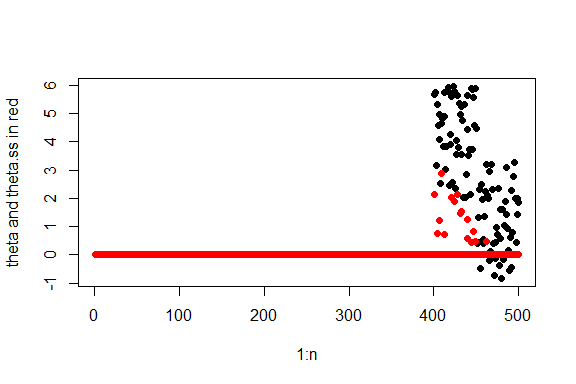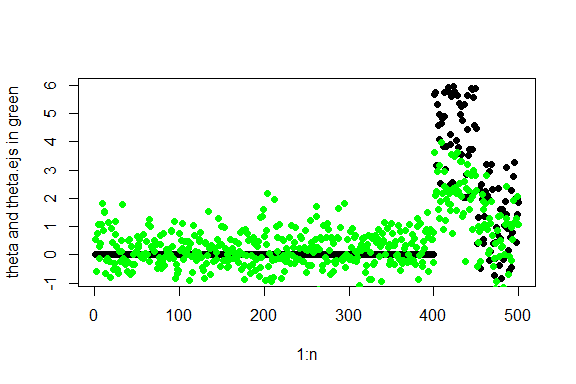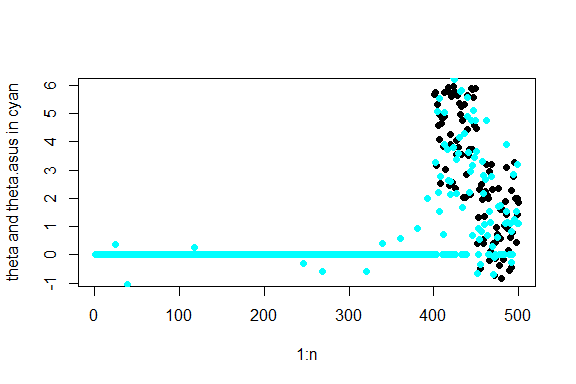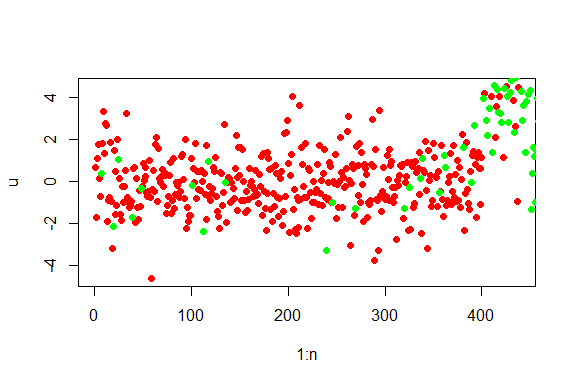# demo-asus

This vignette provides a quick demo of the functionalities of the asus package.

We will consider a two sample estimation problem where the goal is to estimate an $$n$$ dimensional parameter $$\boldsymbol{\theta} =\boldsymbol{\theta}_x-\boldsymbol{\theta}_y$$ based on observations $$X_i\sim N(\theta_{x,i},1)$$ and $$Y_i\sim N(\theta_{y,i},1)$$ where $$i=1,\ldots,n$$.

## Example 1 - SureShrink estimator and SURE estimate of risk

# Parameter of interest
n<-500
set.seed(42)
theta.x<- c(matrix(0,1,400),runif(n-400,2,6))
set.seed(42)
theta.y<- c(matrix(0,1,450),runif(n-450,2,3))
theta<-theta.x-theta.y

#observations
v.x<- rep(1,n)
v.y<- rep(1,n)
set.seed(42)
x<-rnorm(n,theta.x,v.x)
set.seed(84)
y<-rnorm(n,theta.y,v.y)

# SureShrink estimator of theta
library("asus")
u<- x-y
v.u<- v.x+v.y
theta.ss<-sureshrink(u,v.u)$est plot(1:n,theta,pch=19,ylab ="theta and theta.ss in red") points(1:n,theta.ss,col="red",pch=19)# SURE estimate of risk of theta.hat and choice of threshold out<- sureshrink.mse(u,v.u) mse.ss<- out$sure.est
t.ss<- out$t ## Example 2 - Extended James-Stein estimator # EJS estimator of theta theta.ejs<- ejs(u,v.u) plot(1:n,theta,pch=19,ylab ="theta and theta.ejs in green") points(1:n,theta.ejs,col="green",pch=19)## Example 3 - ASUS and SURE estimate of risk of ASUS # side information on theta s<- abs(x+y) out<- asus(u,v.u,s) # ASUS estimator theta.asus<- out$est

plot(1:n,theta,pch=19,ylab ="theta and theta.asus in cyan")
points(1:n,theta.asus,col="cyan",pch=19)# SURE estimate of risk of ASUS
mse.asus<-out$mse # Grouping and thresholding parameters tau<- out$tau
t.asus<- out$t # Group sizes n.asus<- out$size

You will notice that mse.asus = 187.4638917 < 816.9164054 = mse.ss due to the efficient incorporation of side information $$\boldsymbol{s}$$ into the shrinkage estimation procedure.

Finally in the plot above, the green and the red dots represent the two groups constructed by ASUS using the side information in $$\boldsymbol{s}$$.

i1<- (s<=tau)
i2<-(s>tau)
idx<-1:n
plot(idx[i1],u[i1],xlab="1:n",ylab="u",col="red",pch=19)#group 1
points(idx[i2],u[i2],col="green",pch=19)#group 2Notice how the two groups differ with respect to their signal sparsity with group 1 (red) being more sparse than group 2 (green). Indeed, t.asus = 3.4535719 > 0.4808622 = t.asus and compare this with t.ss = 3.5255094.